# Bayesian Merging Scheme

The Strong Aggregating Algorithm for the logarithmic loss function and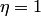is the same as the Bayesian scheme, which goes back to DeSantis et. al 1988, in the case of countable number of experts and number of outcomes. We call it the Bayesian Algorithm (BA) as it is virtually identical to the Bayes rule used in Bayesian learning (the main difference being that the experts are not required to follow any prediction strategies). We consider the case when the set of possible outcomes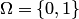and the prediction set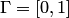.

The Bayesian Algorithm works as follows.

Initialize weights of experts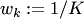,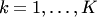.
Initialize cumulative losses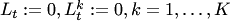.
FORExperts announce predictions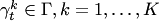.
Predict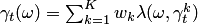.
Reality announces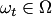.
Update the weights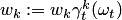and normalize them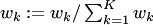,.
END FOR.

The following theoretical bound on the cumulative logarithmic loss of the Bayesian algorithm holds in case of the uniform weights distribution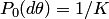.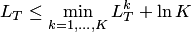for all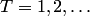.

### Bibliography

• Alfredo DeSantis, George Markowsky, and Mark N. Wegman. Learning probabilistic

prediction functions. In ''Proceedings of the 29th Annual IEEE Symposium on Foundations of Computer Science'', pages 110–119, Los Alamitos, CA, USA, 1988. IEEE Computer Society.